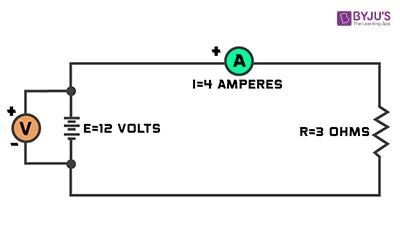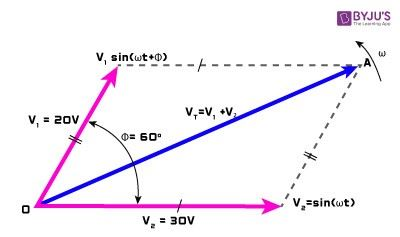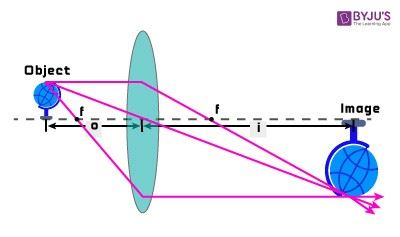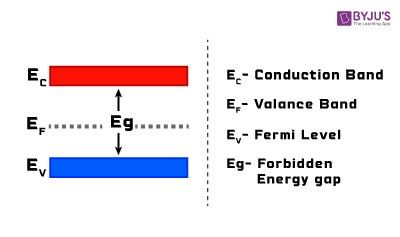Checkout JEE MAINS 2022 Question Paper Analysis : Checkout JEE MAINS 2022 Question Paper Analysis :

# Physics Diagrams

Many children hear the word “physics”, and they run for cover but frankly, the subject is not all that difficult when the basic concepts are understood with the help of physics diagrams. Physics is all around us, which adds as a major benefit to the subject as it creates curiosity in us. Hence, developing an interest in the subject becomes an easy task.

But, the question still arises “Why Diagrams?”

By learning physics diagrams, students can visualise their topics and help them retain the concepts for a long period of time. Learning through diagrams also helps the students to pen down the concept in a better way, resulting to fetch more marks in the exam.

Often in physics, we use free-body diagrams commonly known as FBD or force diagram. These kinds of diagrams are graphical illustrations that are used to visualize the applied forces and movements of a body in a given condition as well as to calculate the resulting reactions. These FBD’s are not meant to be scaled; they are usually drawn as the problem is being solved.

Let us look at few physics diagrams:### Circuit Diagram:

A circuit can be defined as a path in which two or more points along which an electric current can be carried. A circuit diagram is a graphical representation of an electrical circuit. These diagrams show the actual electrical connections of a circuit. A circuit diagram can also have a circuit breaker which is a device that interrupts the path when required to protect other devices that are attached to the circuit.### Schematic Diagram:

A schematic diagram is a representation of the elements of a system using graphic symbols, abstract rather than usual realistic pictures. These diagrams usually omit all the irrelevant information.### Phasor Diagram:

A phasor diagram is a complex number that represents a sinusoidal function whose, angular frequency, amplitude (A), and initial phase are time-invariant. A phasor is a vector that has an arrowhead at one end which signifies partly the maximum value of the vector quantity (V or I) and partly the end of the vector that rotates. It also has terms “lead” and “lag” which is used to indicate the relationship of a waveform to the other with the generalised sinusoidal expression.### Ray Diagram:

A ray diagram is a diagram that traces the path that light takes in order for a person to view a point on the image of an object. On Ray diagrams, the rays (i.e. lines with arrows) are drawn for the incident and reflected rays. These rays are drawn to help trace and calculate the path of waves or particles through a system with regions of varying propagation velocity, reflecting surfaces and absorption characteristics. Under these circumstances mentioned, the rays can either bend, change their direction, or reflect off surfaces.### Energy Band Diagram:

The range of energies that an electron may possess in an atom is known as the energy band. The energy band diagram is a diagram plotting various key energy levels as a function of some spatial dimensions. The energy band diagram SHOULD NOT be confused with the band structure. There are few important energy bands, they are as follows:

• Valence Band
• Conduction Band
• Forbidden Band

Stay tuned with BYJU’S to know more physics diagrams, important physics topics, and experiments.

Test Your Knowledge On Physics Diagrams!